# Lecture - 24 Case Study

# Lecture - 23 Case Study

# Lecture - 22 Verification and Validation

# Lecture - 21 Software Metrics and Quality

# Lecture - 20 Structural Programming and Some implementation

# Lecture - 19 Software Testing - II

# Lecture - 18 Software Testing - I

# Lecture - 16 Class and Component Level Design

# Lecture - 15 Design Patterns

# Lecture - 14 Software Design - Primary Consideration

# Lecture -13 Production Quality Software - Introduction

# Lecture-12 Data Modelling

# Lecture - 11 Data Modelling - ER Diagrams, Mapping

# lecture - 9 Process Modelling - DFD , Function Decomp

# Lecture - 10 Process Modelling - DFD, Function Decomp

# Lecture - 8 Systems Modelling Overview

# Lecture - 7 Algebraic Specification Methods

# Lecture - 6 Formal Specification

# Lecture -5 Requirements Engineering / Specification

# Lecture - 4 Overview of Phases

# Lecture-3 Overview of Phases

# Lecture - 2 Introduction to Software Engineering

# Lecture - 1 Introduction to Software Engineering

# Program in C for multiplication table using while loop

Input :-

Output :-

Code :-

#include <stdio.h>
int main()
{
int n,i=1;
printf("Enter a number :");
scanf("%d",&n);
while (i<=10)
{
printf("%d * %d = %d \n", n, i, n*i);
i++;
}
}

# Print multiplication table in C

Print multiplication table in C

Input :-

Output :-

Code :-

#include <stdio.h>
int main()
{
int n, i;
printf("Enter an integer: ");
scanf("%d",&n);
for(i=1; i<=10; ++i)
{
printf("%d * %d = %d \n", n, i, n*i);
}

return 0;
}

# Program to calculate factorial of a number

Input :-

Output :-

Code :-

#include <stdio.h>

int main()
{
int c, n, fact = 1;

printf("Enter a number \n");
scanf("%d", &n);

for (c = 1; c <= n; c++)
fact = fact * c;

printf("The factorial of %d is = %d\n", n, fact);

return 0;
}

# Program to check a Number is Negative or Positive

Input :-

Outputs : -

Code :-

#include <stdio.h>
#include <stdlib.h>

int main()
{
int a;

printf("Enter a number : \n");
scanf("%d", &a);
if
(a<0)
printf("The number is negative\n");
else if
(a==0)
printf("You Entered Zero\n");
else
printf("The number is positive\n");
return 0;

}

# To check a year is leap or not in C

To check a year is leap or not in C

Input :

Output :

Code :

#include <stdio.h>

int main()
{
int c;
printf("Enter the year :\n");
scanf("%d",&c);
{if ((c%4==0)&&(!(c%100==0)||(c%400==0)))
printf("The year is a leap year\n");
else
printf("The year is not a leap year\n");
}
return 0;
}

# To check whether a number is divisible by 6 and 4

To check whether a number is divisible by 6 and 4

Input :

Output :

Code :

#include <stdio.h>

int main()
{
int c;
printf("Enter a number :\n");
scanf("%d",&c);
{ if ((c%6==0) && (c%4==0))
printf("The number is divisible by 6 and 4\n");
else
printf("The number is not divisible by 6 and 4\n");
}
return 0;
}

# Program to print first 1000 number

Program to print first 1000 number

Input:-

Output:-

Code :-

#include <stdio.h>

int main()
{
int c=1;

while (c <= 1000)

{
printf("%d ", c);
c++;
}

return 0;
}

# To Check whether a number is prime or not

To Check whether a number is prime or not

Input:-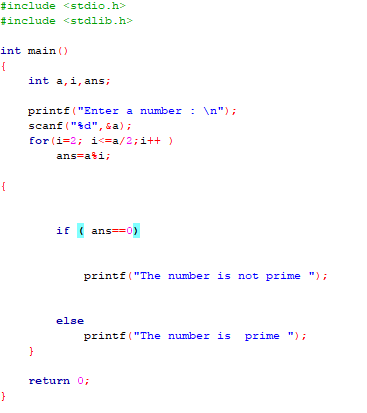Output:-

1)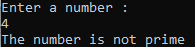2)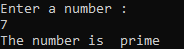Code:-

#include <stdio.h>
#include <stdlib.h>

int main()
{
int a,i,ans;

printf("Enter a number : \n");
scanf("%d",&a);
for(i=2; i<=a/2;i++ )
ans=a%i;

{

if ( ans==0)

printf("The number is not prime ");

else
printf("The number is  prime ");
}

return 0;
}

# Program to check divisibility by 5

Program to check divisibility by 5

Input: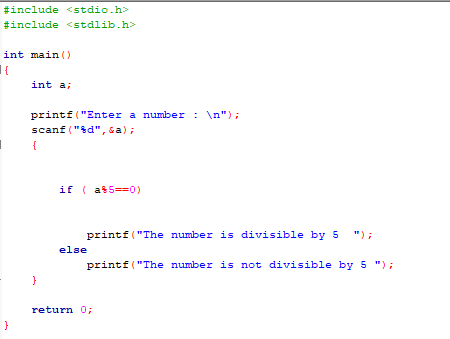Output:

1)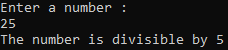2)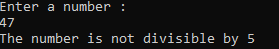Code:

#include <stdio.h>
#include <stdlib.h>

int main()
{
int a;

printf("Enter a number : \n");
scanf("%d",&a);
{

if ( a%5==0)

printf("The number is divisible by 5  ");
else
printf("The number is not divisible by 5 ");
}

return 0;
}

## Categories

Android (21) AngularJS (1) Books (10) C (75) C# (4) C++ (81) Downloads (1) Engineering (13) FPL (17) Hadoop (1) HTML&CSS (38) IS (25) Java (87) Leet Code (4) PHP (20) Python (214) R (69) Software (14) SQL (27)